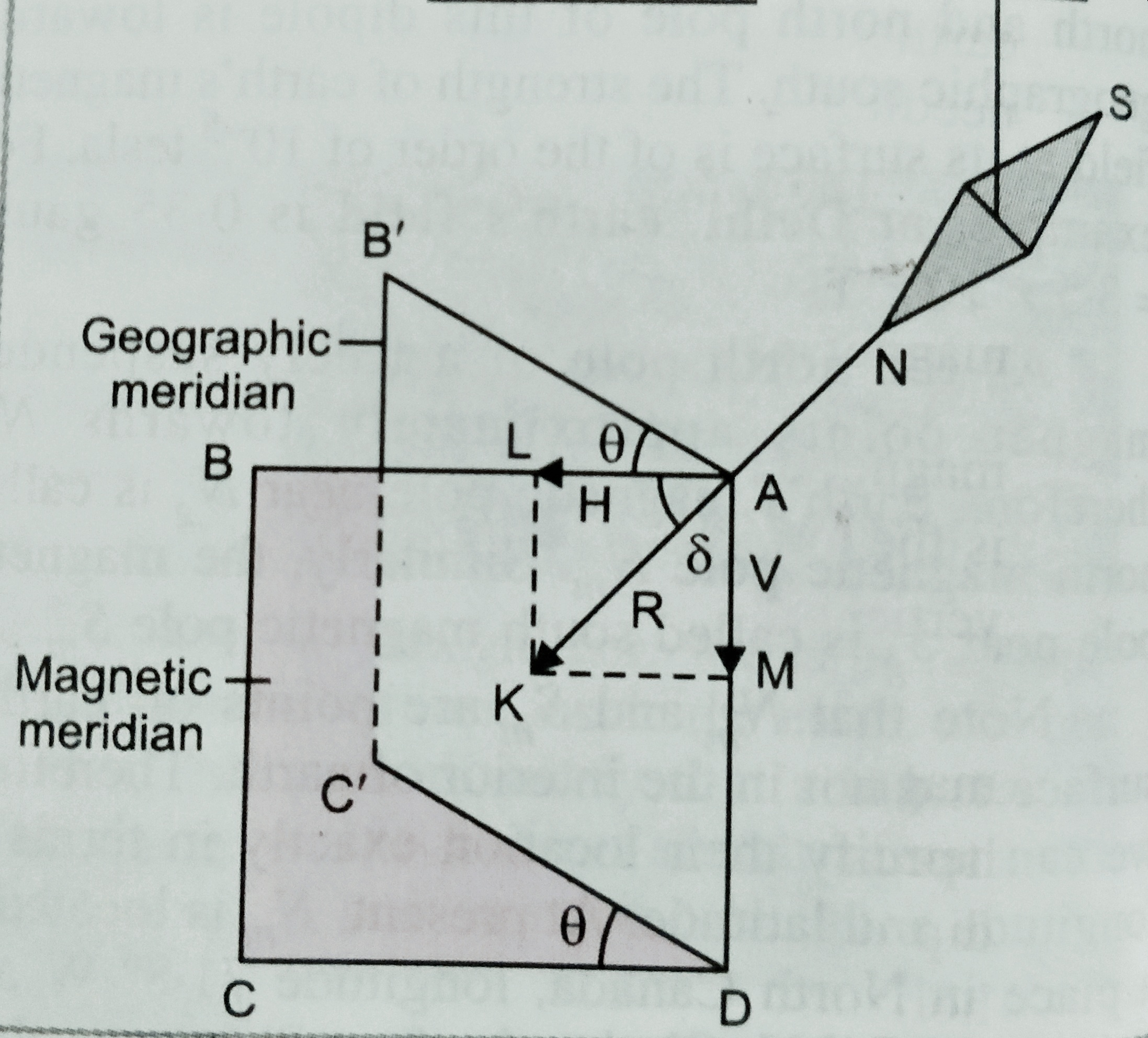Categories

# Magnetic elements of earth

In this topic we will discuss about the magnetic elements of earth. All three kinds of magnetic elements of earth , angle of dip,angle of declination and horizontal components of earth magnetic field.

Magnetic elements of earth

Magnetic elements of earth at a place are the quantities which describe completely the magnitude and direction of magnetic field at that place.

There are three magnetic elements of earth-

(i) Magnetic declination (ϴ);

(ii) Magnetic inclination or angle of dip (ẟ);

(iii) Horizontal components of earth’s magnetic field(H).(i) Magnetic declination (ϴ);-

The small angle between magnetic and geographic axis at a place is known as magnetic declination at that place .

Or, In other words we can define , angle between geographic and magnetic meridian at a place on earth is known as the magnetic declination at that place.

(ii) Magnetic inclination or angle of dip –

Magnetic inclination at a place on the earth is the angle between total strength of magnetic field and horizontal lines in magnetic meridian.

The value of angle of dip at a place on the earth is measured using an instrument called ‘ Dip circle’.

(iii) Horizontal component-

It is the components of total field strength of earth’s magnetic field in the horizontal direction in magnetic meridian.

As shown in figure.  , total field strength is represented by AK and angle <BAK = ẟ

Then the magnetic field along AB ( horizontal) is given as

H = R cos ẟ………..(i)

And magnetic field along AD is given as V= R sin ẟ  ………….(ii)

Squiring and adding equation (i) and (ii) is given as

H2 + V2 = R2 ( Cos2ẟ+ Sin2ẟ) ;

So , R = √( H2 + V2)  …………(III)

Again if we divide  (ii) by (i) we get

R sinẟ/Rcosẟ = V/H

Or,  Tan ẟ = V/H …………………(iv)

[**** important information about angle of dip –

(1). At a place on the magnetic poles , total earth’s magnetic field is perpendicular to the surface of earth .( ẟ=900 )

So, H = R cos 900 = 0

It means R = V  ( total magnetic field is only in vertical direction)

(2) At a place on equator , total magnetic field is parallel to the earth ( ẟ=00)

So , V= Rsin00 = 0

It means R = H ( total magnetic field is in horizontal direction).

(3) In a vertical plane at an angle ϴ with the magnetic meridian.

Then apparent H’ = H cos ϴ

Again, apparent V’ = V,

Therefore tanẟ’ = V’/H’ = V/Hcosϴ = tanẟ/cosϴ   ;   (ẟ’ is the apparent value of angle of dip).

(4) If ẟ1 and ẟ2 are observed angle of dip in two arbitrary vertical planes which are perpendicular to each other then the true angle of dip ẟ is given as –

Cot2ẟ=cot21 + cot22.

(5) If λ is the magnetic latitude at a place, then it can be shown that the angle of dip at that place is given as ,   tanẟ = 2 tan λ …]

## 1 reply on “Magnetic elements of earth”

[…] Magnetic elements of earth […]